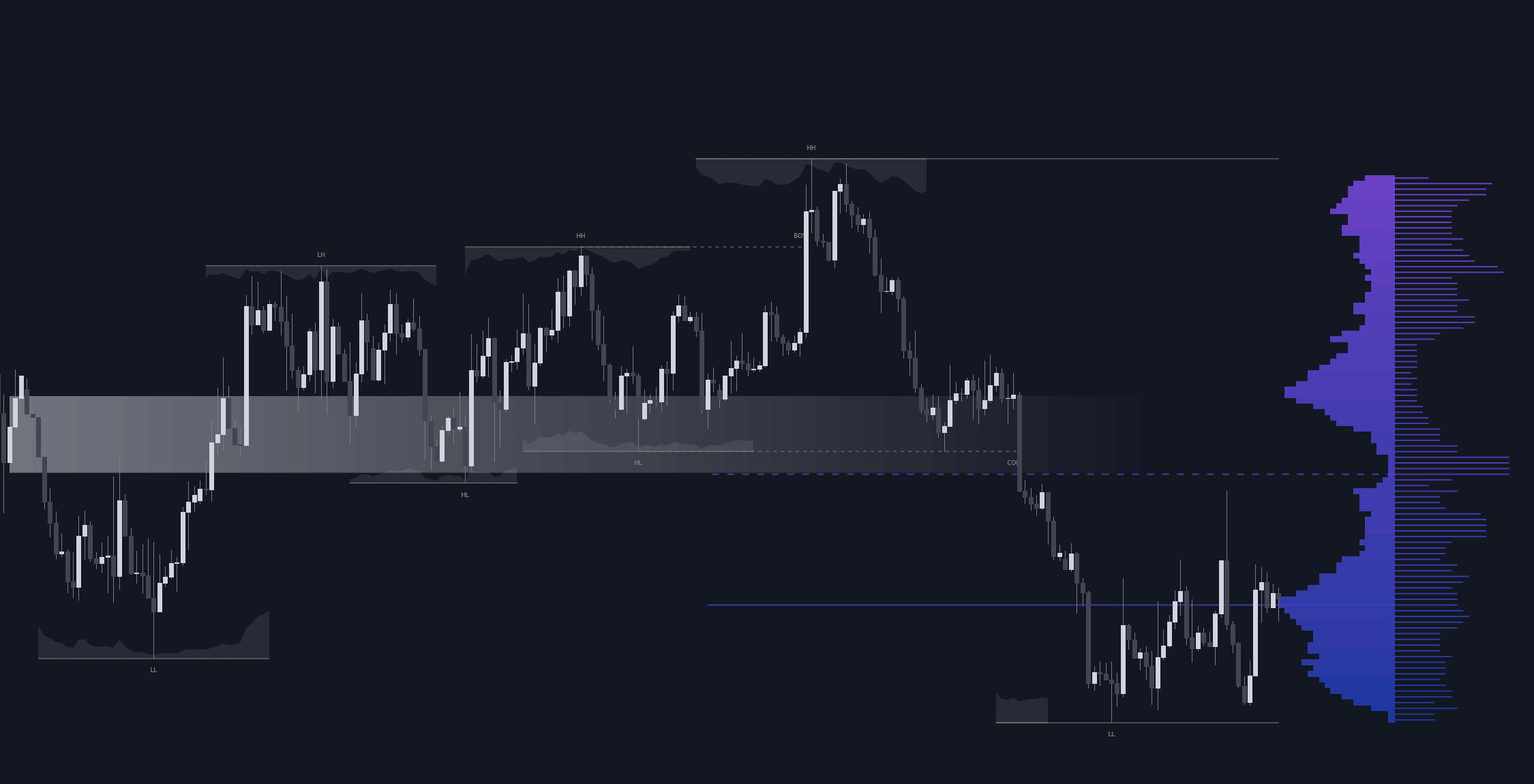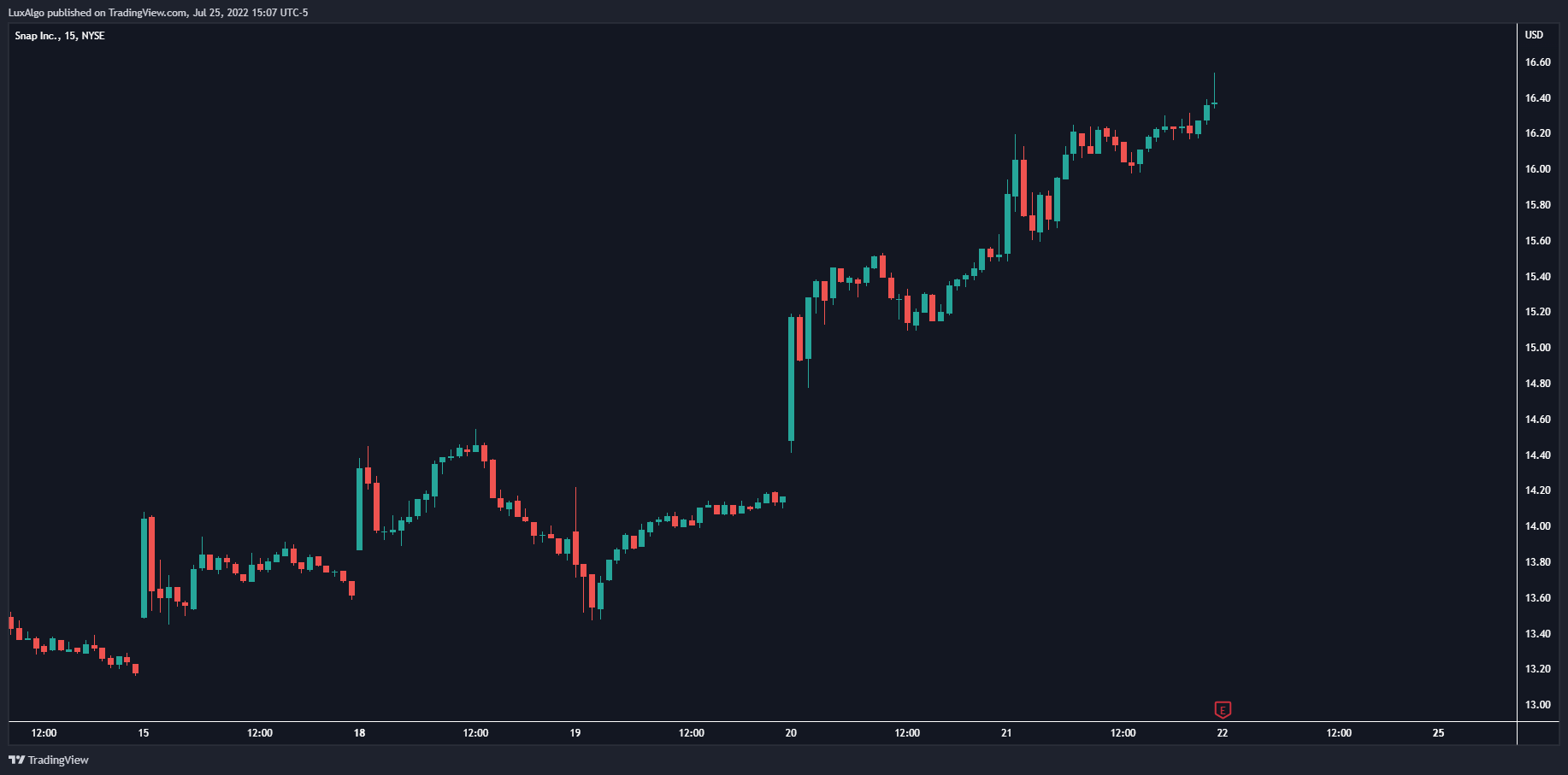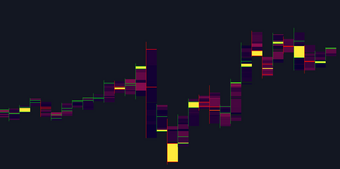# Linear Regression Fan

This indicator displays a fan using a linear regression fit to the price as a base. All lines are equidistant and are drawn from the first point of the linear regression to the most recent point of the linear regression plus the root-mean-square deviation (RMSD) multiplied by a certain factor.

This indicator displays a fan using a linear regression fit to the price as a base. All lines are equidistant and are drawn from the first point of the linear regression to the most recent point of the linear regression plus the root-mean-square deviation (RMSD) multiplied by a certain factor.

### Settings

• Length: Lookback period for the linear regression .
• Mult: Multiplier for the RMSD, allows returning wider fans.
• Lines Per Side: Number of lines on each side of the fan.
• Src: Input source of the indicator.

### Usage

Traders often use the lines of fans to determine significant points of support or resistance at which they might expect price variations to reverse.

The length can be adjusted so that the starting point of the linear regression is located at a pivot high/low.

Some technical analysts use the measure rule of broadening wedges with fans when price breaks one of the extremities. This allows setting precise take-profits/stop-losses.

## Recent posts### Smart Money Concepts (SMC) Explained for Trading Forex, Stocks, & Crypto

##### September 9, 2022### The Best Time Frame for Trading (Actual Truth)

##### August 27, 2022### Liquidity Heatmap LTF

##### May 31, 2022